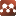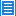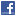## Geometry## A Poincaré-Hopf Type Formula for A Pair of Vector Fields

Authors: Xu Chen

We extend the reslut about Poincar\'e-Hopf type formula for the difference of the Chern character numbers (cf.) to the non-isolated singularities, and establish a Poincar\'e-Hopf type formula for a pair of vector field with the function $h^{T_{\mathbb{C}}M}(\cdot,\cdot)$ has non-isolated zero points over a closed, oriented smooth manifold of dimension $2n$.